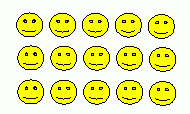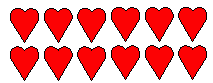Email us to get an instant 20% discount on highly effective K-12 Math & English kwizNET Programs!

#### Online Quiz (WorksheetABCD)

Questions Per Quiz = 2 4 6 8 10

### Grade 3 - Mathematics1.4 Multiplication Pictures

 Method: Count the pictures in each row. Multiply the number of patterns in each row by the total number of rows. Example: What is the multiplication form shown in the figure?This is made of 3 rows of 5 in each, we hence write as 5*3. Answer : 3 * 5 = 15 Directions: Write the multiplication form of the following figures. Also write atleast 5 examples of your own.
 Q 1: What is the multiplication form shown in the figure?5 x 1 = 61 x 5 = 61 x 5 = 5 Q 2: What is the multiplication form shown in the figure?2 x 6 = 142 x 6 = 132 x 6 = 12 Q 3: What is the multiplication form shown in the figure?4 x 3 = 124 x 3 = 134 x 3 = 11 Q 4: What is the multiplication form shown in the figure?6 x 5 = 306 x 4 = 247 x 4 = 28 Q 5: What is the multiplication form shown in the figure?2 x 4 = 102 x 5 = 82 x 4 = 8 Q 6: What is the multiplication form shown in the figure?4 x 2 = 93 x 2 = 84 x 2 = 8 Question 7: This question is available to subscribers only! Question 8: This question is available to subscribers only!

#### Subscription to kwizNET Learning System offers the following benefits:

• Unrestricted access to grade appropriate lessons, quizzes, & printable worksheets
• Instant scoring of online quizzes
• Progress tracking and award certificates to keep your student motivated
• Unlimited practice with auto-generated 'WIZ MATH' quizzes